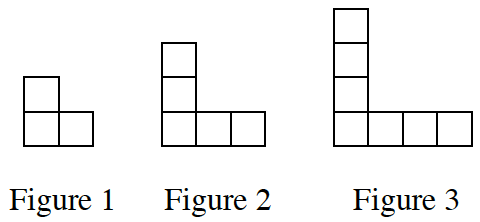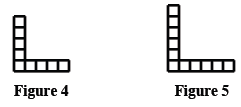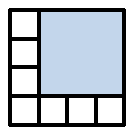Home > ACC7 > Chapter cc31 > Lesson cc31.1.2 > Problem1-12

1-12.

Examine the tile pattern at right. 1-12 HW eTool (CPM). Homework Help ✎1. On your paper, sketch Figures 4 and 5.

Before you can draw the next figures, you must understand the pattern. How many unit squares are added to form the next figure? Where are they added?2. How does the pattern grow? Explain how you know.

What is the difference in number of squares between Figure 1 and Figure 2? Between Figure 2 and Figure 3?

The pattern grows each time by two tiles, one on each side of the figure.

3. How many tiles will there be in Figure 100? Explain how you know.

Try thinking of the pattern not as an 'L' shape, but a square with some part removed. What size would the square for Figure 100 be?$201$ tiles

Use the eTool below to draw the additional figures.
Click the link at right for the full version of the eTool: CC3 1-12 HW eTool